# Mathematics | Set Operations (Set theory)

Union
Union of the sets A and B, denoted by A ∪ B, is the set of distinct element belongs to set A or set B, or both.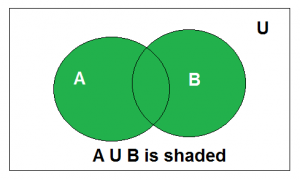Above is the Venn Diagram of A U B.

Example : Find the union of A = {2, 3, 4} and B = {3, 4, 5};
Solution : A ∪ B = {2, 3, 4, 5}.

Intersection
The intersection of the sets A and B, denoted by A ∩ B, is the set of elements belongs to both A and B i.e. set of the common element in A and B.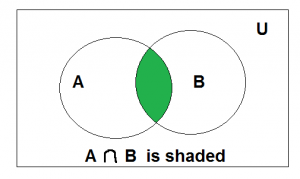Above is the Venn Diagram of A ∩ B.

Example: Consider the previous sets A and B. Find out A ∩ B.
Solution : A ∩ B = {3, 4}.

Disjoint
Two sets are said to be disjoint if their intersection is the empty set .i.e sets have no common elements.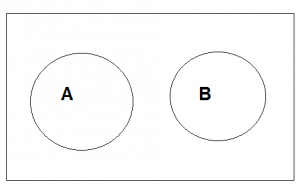Above is the Venn Diagram of A disjoint B.

For Example
Let A = {1, 3, 5, 7, 9} and B = { 2, 4 ,6 , 8} .
A and B are disjoint set both of them have no common elements.

Set Difference
Difference between sets is denoted by ‘A – B’, is the set containing elements of set A but not in B. i.e all elements of A except the element of B.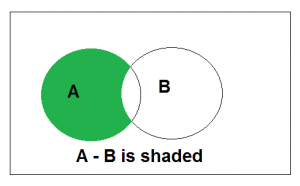Above is the Venn Diagram of A-B.

Complement
Complement of a set A, denoted by, is the set of all the elements except A. Complement of the set A is U – A.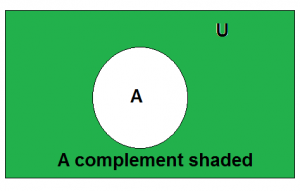Above is the Venn Diagram of Ac

Formula:

1.2.Properties of Union and Intersection of sets:

1. Associative Properties: A ∪ (B ∪ C) = (A ∪ B) ∪ C and A ∩ (B ∩ C) = (A ∩ B) ∩ C
2. Commutative Properties: A ∪ B = B ∪ A and A ∩ B = B ∩ A
3. Identity Property for Union: A ∪ φ = A
4. Intersection Property of the Empty Set: A ∩ φ = φ
5. Distributive Properties: A ∪(B ∩ C) = (A ∪ B) ∩ (A ∪ C) similarly for intersection.

6. Example : Let A = {0, 2, 4, 6, 8} , B = {0, 1, 2, 3, 4} and C = {0, 3, 6, 9}. What are A ∪ B ∪ C and A ∩ B ∩ C ?

Solution: Set A ∪ B &cup C contains elements which are present in at least one of A, B, and C.

A ∪ B ∪ C = {0, 1, 2, 3, 4, 6, 8, 9}.

Set A ∩ B ∩ C contains an element which is present in all the sets A, B and C .i.e { 0 }.

See this for Set Theory Introduction.My Personal Notes arrow_drop_up

Article Tags :

10

Please write to us at contribute@geeksforgeeks.org to report any issue with the above content.